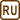## Heat Loss Through a Glass Pane

### Task number: 1277

How much heat is lost within an hour through a pane of glass 4 mm thick and with an area of 2 m2 given that the outdoor temperature is −10 °C and the room temperature is 20 °C?

• #### Hint

What variables does the amount of heat that passes through a pane of glass depend on? Does it depend directly or inversely? The constant of proportionality is called the thermal conductivity coefficient.

• #### Analysis

The heat that passes through the pane of glass per a unit of time is directly proportional to the temperature differences and the surface area of the glass pane, and inversely proportional to the thickness of the glass. The constant of proportionality is the thermal conductivity coefficient.

• #### Numerical values

 d = 4 mm = 4·10−3m the thickness of the glass pane S = 2 m2 the surface area of the glass pane t1 = −10 °C the outdoor temperature t2 = 20 °C the room temperature τ = 3600 s the time, for which we measure how much heat passes through the glass pane Q = ? the heat lost through the glass pane within one hour

From The Handbook of Chemistry and Physics:

 λ = 1,0 W m−1K−1 the thermal conductivity coefficient of glass
• #### Solution

The heat that passes through the glass pane is directly proportional to the surface area S of the pane, the temperature difference Δt and time τ, and inversely proportional to the pane thickness d. The constant of proportionality is the thermal conductivity coefficient λ of the material. Therefore, we can write:

$Q=\frac{\lambda S\tau\mathrm{\Delta}t}{d}=\frac{\lambda S\tau\left(t_2-t_1\right)}{d}\,\mathrm{.}$

Numerical insertion:

$Q=\frac{\lambda S\tau\left(t_2-t_1\right)}{d}=\frac{1.0\cdot{2}\cdot{3600}\left(20+10\right)}{4\cdot{10^{-3}}}\,\mathrm{J}\dot=$ $\dot=54\cdot{10^6}\,\mathrm{J}=54\,\mathrm{MJ}$
• #### Answer

The amount of heat that passes through a glass pane within one hour is approximately 54 MJ.

• #### For information

If we had windows made of glass with properties stated in this task, the heat losses would be enormous. The heat losses are nowadays reduced by using various methods, for example doubling the glass. The layer of air between the two panes has much lower value of the thermal conductivity coefficient than the glass itself, hence loosing less heat. The space between two glass panes can also be filled with other gases, such as argon, xenon or krypton; all of them have lower values of thermal conductivity coefficient.

Another method is using a thin layer of metal coating or bonding a foil to reflect the heat back into the room.

The manufacturers characterize the properties of window panes with the coefficient U0, which indicates, how much heat passes through a window with surface area of 1 m2 at a temperature difference 1 °C per a unit of time. Its unit is therefore Wm−2K−1. We obtain the coefficient U0 by dividing the thermal conductivity coefficient λ by the thickness d of the pane.

For glass from this task we get the value of the coefficient U0:

U0 = 250 W m−2K−1.

The manufacturers of window panes indicate that the value of U0 of certain types of windows is less than 1.15  W m−2K−1, which differs significantly from the value for the glass in our task.